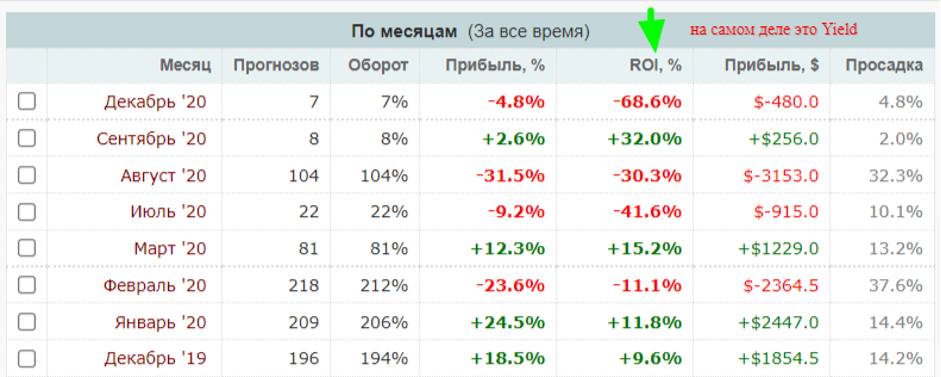# What is ROI in Betting?

30.08.2021

All about the ROI betting metric. What is it, how to calculate potential and real profit using these parameters, how does ROI differ from Yield. Which indicator is better and why. All this is in our today's material.

## How ROI differs from Yield

In Russia, many people confuse the concepts of ROI and Yield. Even the largest Russian-language verifier Bet-hub takes into account a parameter in the ROI graph, which is actually called Yield. Many other resources also often substitute these concepts.Let's figure out the difference once and for all:

1. ROI (return on investment) - return on investment - an indicator of profitability for a potential investor. The profitability is calculated taking into account the amount of investments in the capper project. Calculation formula: ((X - Y) / B) x 100 = ROI. X in this formula is the sum of all payments, Y is all bets made, and B is all investments (or the total bank).

Example 1:

– investor A invested 100 thousand rubles in bettor's bets. Bettor made bets in the amount of 2,000,000 rubles, and received payments of 2,500,000 rubles. What is the ROI? ((2,500,000 - 2,000,000) / 100,000) x 100 = + 500%. That is, the client received a fivefold return on investment at a decent distance, and can count on similar results in the future.

Example 2:

– client B made an investment in the capper rates of RUB 1,000,000. After 1000 bets for a total of 10 million rubles, the payments were only 8 million rubles. We count by the formula: ((8,000,000 - 10,000,000) / 1,000,000) x 100 = -200%. That is, a double drain of the invested money.

1. Yield - an indicator of the efficiency of the player's betting activity, the percentage of profit from the turnover. It takes into account only the turnover of funds and the amount of net profit (loss). Calculation formula: ((X - Y) / Y) x 100 = Yield. Here also X is the sum of all payments, and Y is the sum of the entire turnover of funds.

Example 1:

– player A made 1000 bets for a total of 300,000 rubles, and received payments for 400,000 rubles. Its Yield is: ((400000 - 300000) / 300000) x 100 = + 33.33%. This means that at a distance he receives + 33.3% profit from each of his bets, regardless of the outcome.

Example 2:

– player B made bets totaling 1,000,000 rubles and received payments of 900,000 rubles. Obviously, his Yield will turn out to be negative: ((900,000 - 1,000,000) / 1,000,000) x 100 = -10%. That is, he loses 10% from each bet on average, as the distance shows.

## Which is better, ROI or Yield?

In our opinion, Yield is much more indicative than ROI. In ROI, players have the ability to manipulate the results by changing parameter B (bankroll or entire investment). Yield, on the other hand, is an honest and unbiased indicator that reflects the effectiveness of betting activities. This information is useful both for the capper himself and for his potential investors.

***

However, most of the resources when they write ROI mean exactly Yield.

***

Yield is also easier to compute because it has fewer variables. Yield is a single, integral and understandable number that means both the percentage of profit from each bet and the distance profit from betting activities in general. With its help, you can easily compare the profitability of several tippers, the potential profit from a particular strategy, evaluate your own rates and forecasts for different periods of time. Moreover, neither the bank nor even the used financial strategy matter here.

***

Capper A earned 50,000 rubles from a turnover of 1,000 bets, and capper B earned 100,000 rubles after his thousandth bet.

It would seem that B is cooler? No, because capper A used a turnover of 500,000 rubles, and B - 30,000,000 rubles. If we compare their Yield score, we see that capper A earns + 10% of each bet, and capper B only + 3.33% of the bet. When the players equal in turnover, the profit of the capper A will be three times higher.

***

And one more important point. Both of these indicators (both ROI and Yield) are purely remote. They can be reliably viewed only at a distance, after about a thousandth bet (the more, the more accurate). Short stretches can show any profit - be hyperplus, zero or even minus, but they should not be taken into account. Conclusions can only be made closer to 1000 bets, which is why this number appears in all calculations.